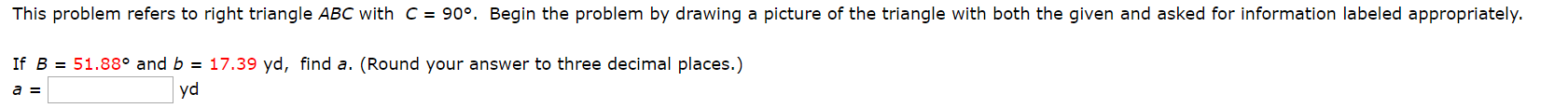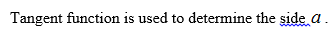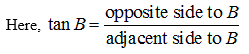# This problem refers to right triangle ABC with c = 90°. Begin the problem by drawing a picture of the triangle with both the given and asked for information labeled appropriately.17.39 yd, find a. (Round your answer to three decimal places.)If B 51.88° and byda = This problem refers to right triangle ABC with c = 90°. Begin the problem by drawing a picture of the triangle with both the given and asked for information labeled appropriately.17.39 yd, find a. (Round your answer to three decimal places.)If B 51.88° and byda =

Question
12 views

Photo attachedhelp_outlineImage TranscriptioncloseThis problem refers to right triangle ABC with c = 90°. Begin the problem by drawing a picture of the triangle with both the given and asked for information labeled appropriately. 17.39 yd, find a. (Round your answer to three decimal places.) If B 51.88° and b yd a = fullscreenhelp_outlineImage TranscriptioncloseThis problem refers to right triangle ABC with c = 90°. Begin the problem by drawing a picture of the triangle with both the given and asked for information labeled appropriately. 17.39 yd, find a. (Round your answer to three decimal places.) If B 51.88° and b yd a = fullscreen
check_circle

Step 1

The diagrammatic representation of the question is shown below.

Step 2Step 3...

### Want to see the full answer?

See Solution

#### Want to see this answer and more?

Solutions are written by subject experts who are available 24/7. Questions are typically answered within 1 hour.*

See Solution
*Response times may vary by subject and question.
Tagged in

### Trigonometry